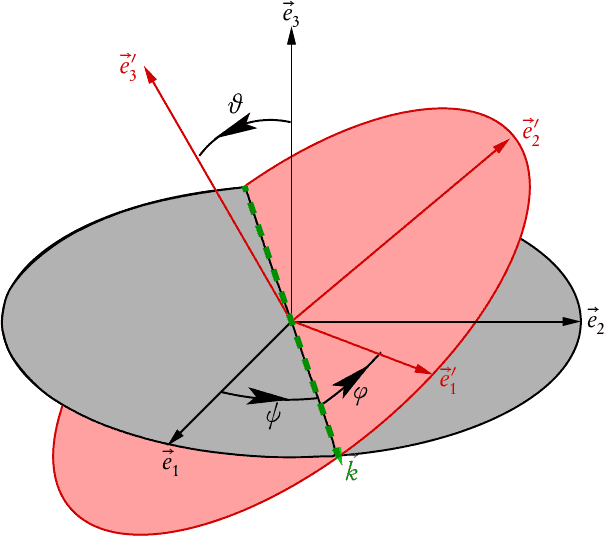# The order of Euler Angle rotations for a top

Trying2Learn
Good Morning All.

I have asked this before, but my post was not clear (my fault: I apologize). I hope this is more clear (please be patient as I try to get to the core of my confusion).

1. In the first figure, below, the spinning top precesses as shown (well, it is not a animated jpg, but it should be clear by reading the text bos)
2. In the second figure, the top nutates
3. Finally, it spins.
(And yes, I know that until there is at least some nutation not zero, one cannot distinguish the precession from the spin, but ignore that due to my poor drawing skills).

In this case, the motions will adhere to Euler angles: 3-axis, then 1, then 3 again)

My question is a general one: upon seeing a spinning top for the first time, how did they decide which motion should be modeled first?

In other words, to demonstrate my stupidity, we apply the rotation matrices as:

PRECESSION MATRIX x NUTATION MATRIX x SPIN MATRIX

But I cannot seem to figure out how they decided they PRECESSION was the first one. I know that one cannot commute rotation matrices, but (and set aside the fact that the upper top is isomorphic to the gyro and set aside that one could use Tait angles) what made them decide on that order and not this order, just by looking at it:

NUTATION MATRIX x PRECESSION MATRIX x SPIN MATRIX

In other words... If I were to be so naive as to model the nutaiton first, then I would have no way to rotate the figure on the right, below, into a model for the precession. I can assume that the body spin is last. This is a lot of handwaving by me, and I am almost comfortable with it, but not really.

#### Attachments

Last edited:

Mentor
Summary:: Euler angles, Gyroscope, top

I cannot seem to figure out how they decided they PRECESSION was the first one.
Precession is first because it is precessing about a fixed axis. Nutation comes second because it nutates about the axis determined by precession. If nutation came before precession you wouldn’t know what axis to nutate about. Spin comes last because you spin about the axis determined by both precession and nutation. That is the only order that makes sense.

•vanhees71
Trying2Learn
Precession is first because it is precessing about a fixed axis. Nutation comes second because it nutates about the axis determined by precession. If nutation came before precession you wouldn’t know what axis to nutate about. Spin comes last because you spin about the axis determined by both precession and nutation. That is the only order that makes sense.

I know I will sound like a pain, but I am not. I thought of what you are saying.

However isn't your statement an "observation?" rather than a foundation to begin constructing a model?

If so, can you transform your statement into a "plan of attack."

Namley: if you saw a top spinning, what would you assert is the first rotation?

Unless! You seem to be saying the following (where I ignore steady precession): yes, the top continues to nutate over to the side, but the axis about which that behavior happens is not constant (it is, well, for want of a better word... precessing). So it is reasonable to assume the model we create must FIRST describe the precession, because all other rotations are rotated from that?

Gold Member
2022 Award
The Euler angles are parametrizing a rotation matrix ##D_{jk}## expressing the the body-fixed Cartesian basis ##\vec{e}_{j}'## in terms of the space-fixed basis (Einstein summation convention applies)
$$\vec{e}_{j}'=\vec{e}_k D_{kj}.$$In the above figure we first rotate around the space-fixed three-axis to turn the space-fixed one-axis into the direction of line of node ##\vec{k}## (Euler angle ##\psi##), which is the intersection of the space-fixed (12)- and the body-fixed (1'2') planes. Then you rotate around the line of node, which is the new 1''-axis, by the Euler angle ##\vartheta## such that the 3-axis is turned into the 3' axis. Finally we rotate around this 3' axis by the Euler angle ##\varphi## which turns the line of node into the body-fixed 1' axis. So we have
$$\hat{D}=\hat{D}^{(3)}(\psi) \hat{D}^{(1)}(\vartheta) \hat{D}^{(3)}(\varphi).$$

•hutchphd, wrobel and Dale
Mentor
However isn't your statement an "observation?" rather than a foundation to begin constructing a model?
I don’t understand. Isn’t “observation” the foundation to begin constructing all models?

•vanhees71
Trying2Learn
I don’t understand. Isn’t “observation” the foundation to begin constructing all models?
Yes, and that is exactly what I wanted to hear someone else confirm

I know it sounded strange, and in the last few hours I worked it all out to convince myself, but I wanted to hear someone say it (even though, now, it is all obvious to me).

Thanks all.

•vanhees71 and Dale
See pic at #4:
Consider 4 frames:
(I) -- ##e_1,e_2,e_3## -- the lab frame
(II) -- ##k,e_3,k\times e_3## -- this frame rotates relative (I) with angular velocity ##\dot\psi e_3##
(III) -- ##k,e_3',k\times e_3'## this frame rotates relative (II) with angular velocity ##\dot\theta k##
(IV) -- ## e_1'e_2'e_3'## this frame rotates relative (III) with angular velocity
##\dot\varphi e_3'##

so the angular velocity of (IV) relative (I) is
$$\dot\psi e_3+\dot\theta k+\dot\varphi e_3'$$
this is an infinitesimal version of the argument from #4

Last edited:
•vanhees71
•